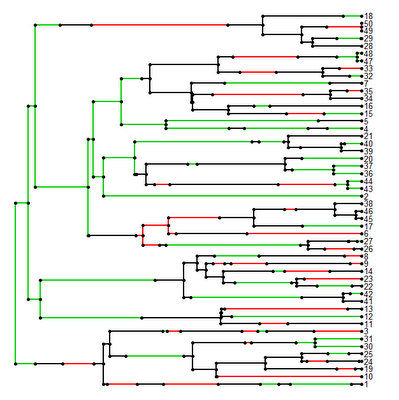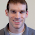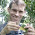## Friday, July 1, 2011

### Small update to make.simmap()

I just posted a small update to my stochastic character mapping function, make.simmap(). The update fixes an error that arises when the tree has any edges that are exactly zero in length. Basically, the issue arose because I programmed the mapping along branch lengths as follows. For estimated transition matrix Q, I first set t to zero, and then I added random deviates from the exponential distribution with rate parameter -Qii (updating i for each state change in the character) while t is less than the total length of the current edge. Unfortunately, this does not work if the length of an edge is exactly zero. In addition, in this case there can be no change in state along the edge, and consequently we can just set our mapped state on that edge to be the sampled state at either the parent or daughter node (which our method of sampling node states guarantees will be the same).

The error in the previous version of make.simmap() will be revealed by the following code:

> require(phytools)
> require(geiger)
> tree<-birthdeath.tree(b=1,d=0,taxa.stop=50)

(birthdeath.tree(...,taxa.stop=N) stops the tree simulation at the moment that the Nth species appears, thus resulting in a pair of zero length terminal edges.)

> q<-list(matrix(c(-1,0.5,0.5,0.5,-1,0.5, 0.5,0.5,-1),3,3))
> x<-sim.char(tree,model.matrix=q,model="discrete")[,,1]
> mtree<-make.simmap(tree,x)
Error in if (names(map)[length(map)] == node.states[i, 2]) accept = TRUE :
argument is of length zero

However, if we load the new version of make.simmap(), the problem goes away:

> source("make.simmap.R")
> mtree<-make.simmap(tree,x)
> plotSimmap(mtree)It works!

This updated version of make.simmap() will be in the next version of "phytools."

Of course, this does not deal with the issue of two taxa with zero evolutionary divergence that have different states. This will also cause an error in make.simmap() but this is more difficult to resolve because, in fact, two species with zero divergence should not have different phenotypes - and the probability that they do under our models will thus always be zero.

1.Hello,

I'm running into a problem trying to use this function. I have a tree with 47 tips and a character with 4 states. When I try this function, I get this warning:

Warning message:
In sqrt(diag(solve(h))) : NaNs produced

and the resulting tree looks all funky: http://d.pr/HTZy

any ideas? don't know if it might be my data, but it seems to run smooth in simmap 1.0. Any insights would be very helpful! Thanks!

2.Hi Rafa.

Yes, I think the problem is due to having too many states (and not enough tip data) to fit the "SYM" model, in which each reversible transition (e.g., 0<->1, 0<->2, and 1<->2) gets a different rate. I encountered this issue when I tried to fit a character with 6 states on an 85 taxon tree. Try fitting the "ER" model (equal rates for all different transition types) instead:

> mtree<-make.simmap(tree,x,model="ER")

Hope this solves the problem! Please report back.

Best, Liam

3.thank you so much for the feedback, Liam. Indeed, changing the model dealt with the warning, thank you so much for that insight. So that got make.simmap working, cool! Thanks!

However, the tree still doesn't look right when I use plotSimmap. The states arriving at the tip are what I'd expect, but there's the tangled thing going on:

http://d.pr/C8bu

(at least I figured out that the "invisible branches" were because my states ranged from 0-3 instead of 1-4).

any ideas? Thanks again!

4.Hmmm. I can't replicate the error. Try the following as it might give me a hint as to what is wrong:

> mtree<-make.simmap(tree,x,model="ER")
> plotSimmap(mtree)

Let me know if this is still all messed up.

Thanks! - Liam

5.thanks again! the examples are indeed working fine. I'll email you the tree and the data, in case you want to try and replicate it. many thanks!

6.I just noticed that in my tree, plotSimmap is plotting the taxa in the exact order (from bottom to top) in which the tree\$tip.label is, which I guess is not the same as they appear in the topology. In the simulated example, the topology sequence of tips and tree\$tip.label are identical. Is there any way I can adjust my tree or something to adjust this? I tried the reorder.phylo cladewise/prunewise but that doesnt' seem to do it... Once again, thanks for the help and the attention, cheers!

7.You're right. I noticed that too. I thought that reorder.phylo() would create that order, but it does not (although read.tree() does - which is how my workaround works). Don't worry, I'll fix this. (Probably tomorrow though.) Thanks for finding this bug!

8.Hi Liam - When using the morphology model in SIMMAP it requires the user to specify priors on the branch lengths (rate parameter) which can be a gamma distribution or a branch length prior and on the bias parameter which is usually a beta distribution or fixed 2-state prior or equal (1/k) prior. Your code doesn't require either of these so how are these parameters being incorporated? I am guessing you automatically use a branch length prior as that makes the most sense for most studies but I am unsure about the bias parameter.

Thanks!

9.Hi.

Yes, in this way it differs from SIMMAP (evidently). In make.simmap(), I have merely fixed these parameters (the parameters of the substitution matrix) at their MLEs. The posterior sample of character histories is then conditioned on these MLEs. Since my function uses ace() in the "ape" package to compute the conditional probabilities, you can specify any substitution model implemented within ace().

The difference here is that Bollback's program, SIMMAP, samples over uncertainty in both the substitution process and the character histories. This is better, obviously, but not simple to do properly (see http://www.simmap.com/pgs/priors.html). I will try to build this into a later, greater version of the function.

Thanks for the question! - Liam

10.Hi Liam,

I am trying to play with your make.simmap function, but I found that in all the simulations, colnames(tree\$mapped.edge) show a different order. This is problematic when I want to combine the results for models fitted over the multiples trees as done for instance with OUwie package.

1.